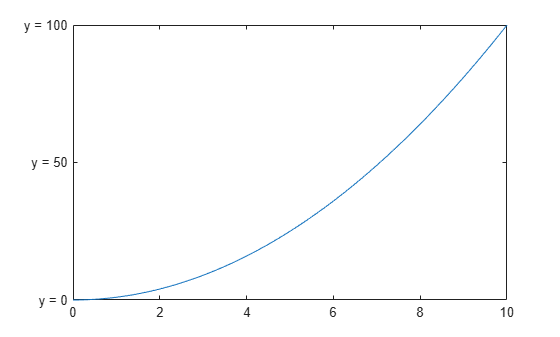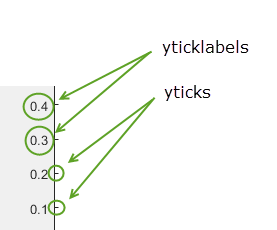# yticks

Set or query y-axis tick values

## Syntax

``yticks(ticks)``
``yt = yticks``
``yticks('auto')``
``yticks('manual')``
``m = yticks('mode')``
``___ = yticks(ax,___)``

## Description

example

````yticks(ticks)` sets the y-axis tick values, which are the locations along the y-axis where the tick marks appear. Specify `ticks` as a vector of increasing values; for example, `[0 2 4 6]`. This command affects the current axes.```
````yt = yticks` returns the current y-axis tick values as a vector.```

example

````yticks('auto')` sets an automatic mode, enabling the axes to determine the y-axis tick values. Use this option if you change the tick values and then want to set them back to the default values. ```
````yticks('manual')` sets a manual mode, freezing the y-axis tick values at the current values. Use this option if you want to retain the current tick values when resizing the axes or adding new data to the axes.```
````m = yticks('mode')` returns the current y-axis tick values mode, which is either `'auto'` or `'manual'`. By default, the mode is automatic unless you specify tick values or change the mode to manual.```

example

````___ = yticks(ax,___)` uses the axes specified by `ax` instead of the current axes. Specify `ax` as the first input argument for any of the previous syntaxes.```

## Examples

collapse all

Create a line plot. Display tick marks along the y-axis at the values 0, 50, and 100. Then, specify a label for each tick mark.

```x = linspace(0,10); y = x.^2; plot(x,y) yticks([0 50 100]) yticklabels({'y = 0','y = 50','y = 100'})```Display tick marks along the y-axis at nonuniform values between 0 and 25. MATLAB® labels the tick marks with the numeric values.

```x = linspace(-5,5); y = x.^2; plot(x,y) yticks([0 2 4 6 8 10 15 25])```Display tick marks along the y-axis at increments of 25, starting from 0 and ending at 100.

```x = linspace(0,10); y = x.^2; plot(x,y) yticks(0:25:100)```Create a stem chart and specify the y-axis tick values. Then, set the y-axis tick values back to the default values.

```stem(1:10) yticks([0 5 10])````yticks('auto')`Starting in R2019b, you can display a tiling of plots using the `tiledlayout` and `nexttile` functions. Call the `tiledlayout` function to create a 2-by-1 tiled chart layout. Call the `nexttile` function to create the axes objects `ax1` and `ax2`. Plot data into each axes. Set the y-axis ticks for the lower plot by passing `ax2` as the first input argument to the `yticks` function.

```tiledlayout(2,1) ax1 = nexttile; plot(rand(3)) ax2 = nexttile; plot(rand(3)) yticks(ax2,0:.2:1)```Remove the tick marks along the y-axis by specifying the tick values as an empty array.

```x = linspace(0,10); y = sin(x); plot(x,y) yticks([])```## Input Arguments

collapse all

Tick values, specified as a vector of increasing values. If you do not want tick marks along the y-axis, specify an empty vector `[]`.

You can specify the tick values as numeric, categorical, datetime, or duration values. However, the type of values that you specify must match the type of values along the y-axis.

Example: `yticks([pi 2*pi 3*pi 4*pi])`

Example: `yticks(0:10:100)`

Example: `yticks([])`

Note

To specify the tick labels, use the `yticklabels` function.

Data Types: `single` | `double` | `int8` | `int16` | `int32` | `int64` | `uint8` | `uint16` | `uint32` | `uint64` | `categorical` | `datetime` | `duration`

Target axes, specified as an `Axes` object or an array of `Axes` objects.

If you do not specify this argument, then `yticks` modifies the current axes.

## Output Arguments

collapse all

Current tick values, returned as a vector.

Current mode, returned as one of these values:

• `'auto'` — Automatically determine the y-axis tick values.

• `'manual'` — Use manually specified y-axis tick values.

collapse all

### Tick Values

The tick values are the locations along the y-axis where the tick marks appear. The tick labels are the labels that you see next to each tick mark. Set the tick values using the `yticks` function. Set the corresponding tick labels using the `yticklabels` function.## Algorithms

The `yticks` function sets and queries several axes properties related to the y-axis tick values.

• `YTick` — Property that stores the y-axis tick values.

• `YTickMode` — Property that stores the y-axis tick value mode. When you set the y-axis tick values, this property changes to `'manual'`.

## Version History

Introduced in R2016b